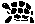# Lookup Tables

This project contains two command line tools for generating C code implementing lookup tables. One is for integer keys, the other for string keys.

An good example is implementing Unicode support: you typically need a lot of lookup tables for sparse, non-contiguous integer sets. With mkhashtable, you create a hash table easily and get a compact and fast static hash table without much hassle.

## Introduction

### Integer Hashing: mkhashtable

The tool for generating integer lookup tables follows a similar idea as gperf, generating a hash table, but the input keys are no strings, but integers.

If you have a set of integers you want to lookup and/or map to other values, i.e., you need an integer dictionary, this is your tool. This is especially true if the integer set is non-contiguous.

mkhashtable is a C++ application that pre-computes a two-bucket cuckoo hash table from a set of integers. The resulting table is very compact (typically the utilisation is 80%), it can be linked statically with your program, and lookup is very fast, the worst case is O(1) with maximally two hash operations.

Further, computing the hash table is fast, too, and the tool allows tuning the generation algorithm for very large sets, trading generation speed for table utilisation as needed.

Cuckoo hash tables have been shown to perform very well on modern processors with caches, because they get rid of the heap-wide distributed linked lists usually used by chaining hashing methods. Instead, all keys and values are stored in one contiguous block of memory.

Future versions of mkhashtable will allow generation of other types of cuckoo hash tables with different numbers of buckets and hash functions, to squeeze the tables even more (trading for lookup speed).

### String Switch: mkstringswitch

If you need a string dictionary, then mkstringswitch is just your tool: it is similar to gperf, taking a specification and generating C code, but the technique for lookup is different: instead of finding a hash function, mkstringswitch uses switch() + memcmp/strcmp to recursively match the strings.

You can use this for very large sets if gperf takes a long time to compute a solution, or for small sets if you forgot how to use gperf and want to get code quickly.

## Examples

### Integer Hash Example

If the input file looks like this:

```100
300
120
100043
2031
102
```

The output file might look like this:

```static map_t map_a= {
300,
100,
102,
};
static map_t map_b= {
2031,
120,
100043,
};
static int hash_a (int x) {
return (((1544301215 * x) - (x ^ 616423921)) % 3);
}
static int hash_b (int x) {
return (((1521445927 * x) - (x ^ 1711634687)) % 3);
}
static map_t const *lookup (int x)
{
map_t const *result;
int index;
index= hash_a (x);
assert (index >= 0 && index < 3);
result= &map_a[index];
if (MAP_KEY_MATCH(result,x))
return result;
index= hash_b (x);
assert (index >= 0 && index < 3);
result= &map_b[index];
if (MAP_KEY_MATCH(result,x))
return result;
return NULL;
}
```

As you can see, some definitions are missing, so if including this in your code, you can do it like this:

```#include <assert.h>
typedef int map_t;
#define MAP_KEY_MATCH(X,Y) *(X) == (Y)
#include "inthash.inc"
```

You can add prefixes to the C identifiers with the --prefix option.

The program has many options, including a 2-dimensional key option where two integers are hashed instead of one.

The above code only implements a boolean set, but you can also map the keys to values, of course, adding a colon after the key in the input file and possibly using the --compose option to put both key and value in the map_t struct (the key might be needed to implement MAP_KEY_MATCH).

### String Switch Example

If the input file looks like this:

```testfoo:  return T_TESTFOO
testbar:  return T_TESTBAR
foobar:   return T_FOOBAR
```

The output file looks like this:

```switch (len) {
case 6:
if (memcmp(c+0, "foobar", 6) == 0) {
return T_FOOBAR;
}
break;
case 7:
if (memcmp(c+0, "test", 4) == 0) {
switch (c) {
case 'b':
if (c == 'a') {
if (c == 'r') {
return T_TESTBAR;
}
}
break;
case 'f':
if (c == 'o') {
if (c == 'o') {
return T_TESTFOO;
}
}
break;
}
}
break;
}
```

You can use this in your own code by wrapping a small function around it:

```MyOwnType lookup_string (char const *c, size_t len)
{
#   include "strswitch.inc"
return T_UNKNOWN;
}
```

mkhashtable-1.89198.tgz
mkhashtable-1.89198.tgz.md5
mkhashtable-2023-01-17.tgz

LibError is required for compilation before v2023-01-17:

liberror-2.1.89882.tgz
liberror-2.1.89882.tgz.md5

## Changes

• ### Version 2023-01-17

• Clean-up: rewrite in C
• Clean-up: remove dependency on liberror and Erwin
• Clean-up: update license to GPLv3
• ### Version 1

• Initial release.

## Index

January 17th, 2023
Comments? Suggestions? Corrections? You can drop me a line.
zpentrabvagiktu@theiling.deDatenschutz